# 1476 Mystery

### Today’s Puzzle:

To solve this mystery puzzle, first, gather some facts. I mean, common factors:
Common Factors of 6, 3, 12, and 9 are 1 and 3.
For 20 and 10 we have 2, 5, and 10.
And for 20 and 60, we can only use 5 or 10 because common factor, 20, is too big for a 1 to 12 puzzle.

Which common factors “Done it”? Don’t jump to conclusions. Remember, a good detective will use the facts and logic to figure out the mystery. Good luck!### Factors of 1476:

• 1476 is a composite number.
• Prime factorization: 1476 = 2 × 2 × 3 × 3 × 41, which can be written 1476 = 2² × 3² × 41
• 1476 has at least one exponent greater than 1 in its prime factorization so √1476 can be simplified. Taking the factor pair from the factor pair table below with the largest square number factor, we get √1476 = (√36)(√41) = 6√41
• The exponents in the prime factorization are 2, 2, and 1. Adding one to each exponent and multiplying we get (2 + 1)(2 + 1)(1 + 1) = 3 × 3 × 2 = 18. Therefore 1476 has exactly 18 factors.
• The factors of 1476 are outlined with their factor pair partners in the graphic below.Simplifying √1476 doesn’t have to be a mystery! Here’s one strategy to do it: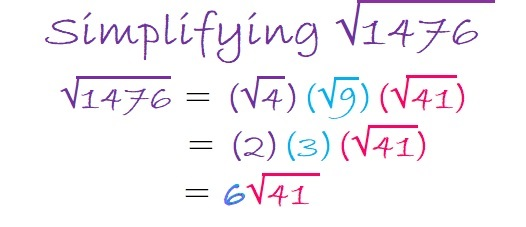### More Facts about the number 1476:

1476 is the sum of two squares:
30² + 24² = 1476

1476 is the hypotenuse of a Pythagorean triple:
324-1440-1476 which is 36 times (9-40-41),
and can also be calculated from 30² – 24², 2(30)(24), 30² + 24².

# 671 is the Magic Sum of an 11 x 11 Magic Square

6 – 7 + 1 = 0 so 671 is divisible by 11.

671 is the sum of the fifteen prime numbers from 17 to 73.

Because 61 is one of its factors, 671 is the hypotenuse of the Pythagorean triple 121-660-671. The greatest common factor of those three numbers practically jumps out at me. Does it do the same thing to you?

Best of all 671 is the magic sum of an 11 x 11 magic square. (That link from wikipedia helped me construct this square. I’ll give directions so you can do it, too!)Notice how every row, column, and diagonal on the square sums to 671. The reason it is the magic sum is because the sum of all the numbers from 1 to 121 can be computed and then divided by 11 (the number of rows). Here is the equation:

• 671 = 121 x 120/2/11

Because 11 is an odd number there are simple directions to complete the entire square:

The number 1 is located in the exact center of the top row.

Find the number 2 on the square. (It’s located on the bottom row just right of the exact center square.) Notice that the numbers 3, 4, 5, and 6 are on the same diagonal. If you imagine the diagonal wrapping around the square, you can continue to follow it for numbers 7, 8, 9, 10, and 11. We can’t put the number 12 along the same diagonal because the number 1 is already in that spot, so we put the 12 UNDER the 11 and begin working on a new diagonal.

Anytime a number already occupies a space on a diagonal, put the next number under the preceding number and continue making a new diagonal. When a diagonal reaches the edge of the square, imagine that edge is connected to the opposite edge and continue the diagonal from the opposite edge.

I found it to be the trickiest placing the numbers 67 and 68, but other than that it was rather easy to know where to put the numbers.

Notice that the difference between any smaller number and the larger number just below it is either 12 or 1.

If you have excel on your computer, click on 12 Factors 2015-11-02, select the magic square tab, and then you can make this 11 x 11 magic square yourself. As you type in numbers, the columns, rows, and diagonals will automatically keep a running sum.

Once you get the square to give the magic sum in each direction, you can try doing the same thing with the 13 x 13 magic square that I’ve included on the same page. Its magic sum is 1105 which can be also be computed:

• 1105 = 169 x 170/22/13.

There is actually many more possible and probably more complicated 11 x 11 and 13 x 13 magic squares. I hope you enjoy making some with this easy method.

—————————————————————————————————

• 671 is a composite number.
• Prime factorization: 671 = 11 x 61
• The exponents in the prime factorization are 1 and 1. Adding one to each and multiplying we get (1 + 1)(1 + 1) = 2 x 2 = 4. Therefore 671 has exactly 4 factors.
• Factors of 671: 1, 11, 61, 671
• Factor pairs: 671 = 1 x 671 or 11 x 61
• 671 has no square factors that allow its square root to be simplified. √671 ≈ 25.903668.—————————————————————————————————

# How to Simplify √450

What’s easier – dividing a number by 2 twice or dividing by 4 once? Most people would agree that dividing by a single digit number like 9 one time is easier than dividing by 3 two times. It also cuts the chance of making a mistake in half.

To simplify square roots, I’ve modified the cake method to look for some specific SQUARE factors rather than beginning with ALL of its prime factors.

Divisibility tricks let me know which square factors to try. I always divide out easy and very common perfect squares 100, 4, 9, and 25 first. (About 82% of reducible square roots are divisible by 4 and/or 9.) Once any of those that apply have been divided out, I look to see if 6 or 10 can be divided out because its easier to divide by 6 or 10 once than to divide by 2 and then by 3 or 5. Only after I have divided out those very easy divisors will I look to divide out any remaining prime factors 2, 3, 5, 7, 11, and so forth. Let me demonstrate this method to simplify √450.

• 450 doesn’t end in 00, so it’s not divisible by 100.
• The last two digits, 50, are not divisible by 4, so 450 is not divisible by 4.
• 4 + 5 + 0 = 9, so 450 is divisible by 9. Therefore, I do a simple division problem, 450 ÷ 9 = 50, leaving room on the page to do any other needed division problems above it.
• The previous quotient, 50, is not divisible by 9, but any number ending in 25, 50, or 75 is divisible by 25, so I divide it by 25 and get 2. Now I am finished dividing.
• The numbers on the outside of the cake are 9, 25, and 2. I take the square root of each of those numbers and get 3, 5, and √2. The product of those square roots is 15√2. Thus √450 = 15√2.Most people have been taught to use a factor tree to find square roots. This is probably 450’s most common factor tree:I like how much more compact and clear this modified cake method is instead. It may take some practice to get used to it, but I will show more examples of it in the future to make it more familiar.

The following puzzle doesn’t have anything to do with the number 450 except it’s the number I gave it to distinguish it from every other puzzle I make. I put the factors of 450 immediately after the puzzle to separate the puzzle from its solution that I add to the post the next day.Print the puzzles or type the factors on this excel file:  12 Factors 2015-04-06

——————————————————————————————————————-

• 450 is a composite number.
• Prime factorization: 450 = 2 x 3 x 3 x 5 x 5, which can be written 450 = 2 x (3^2) x (5^2)
• The exponents in the prime factorization are 1, 2 and 2. Adding one to each and multiplying we get (1 + 1)(2 + 1)(2 + 1) = 2 x 3 x 3 = 18. Therefore 450 has exactly 18 factors.
• Factors of 450: 1, 2, 3, 5, 6, 9, 10, 15, 18, 25, 30, 45, 50, 75, 90, 150, 225, 450
• Factor pairs: 450 = 1 x 450, 2 x 225, 3 x 150, 5 x 90, 6 x 75, 9 x 50, 10 x 45, 15 x 30 or 18 x 25
• Taking the factor pair with the largest square number factor, we get √450 = (√225)(√2) = 15√2 ≈ 21.2132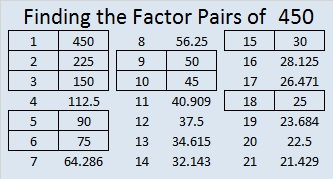——————————————————————————————————————-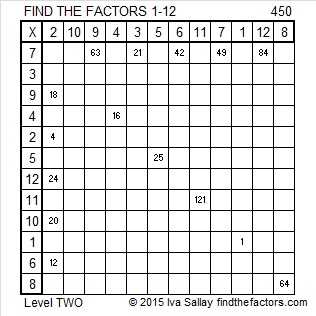# How to Simplify √432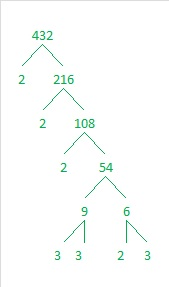Many people use factor trees and prime factorizations to figure out how to simplify square roots. I don’t. I don’t see the point in breaking something completely apart just to put it back together again, especially when it ISN’T necessary to break it completely apart.

So instead of using a factor tree, I’ve modified the cake method, and I use it to simplify square roots.

For example, to find the square root of 500, I would never use its prime factorization: 500 = 2 x 2 x 5 x 5 x 5. Instead, I would first divide 500 by perfect square 100 to get 5. Then I would take the square root of both 100 and 5 to get 10√5.

Only 1% of numbers are divisible by 100, but for the ones that are, I always start by dividing by 100.

Now get this: Roughly 82.5% of all numbers that have simplifiable square roots can be evenly divided by perfect squares four and/or by nine. It is so easy to tell if a number can be evenly divided by either of those numbers. That is why I always start with one hundred, then four, then nine.

Let’s look at 432: Since the number formed from the last two digits, 32, is divisible by 4, I know that 432 is also divisible by four, so I will go ahead and do the division.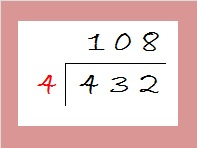Now I look at 108. The last two digits, 08, can also be evenly divided by 4, so I do that division as well: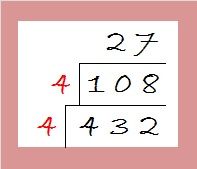108 divided by 4 gives us 27 which is not divisible by 4. However since 2 + 7 = 9, I know that 27 is divisible by 9, so I do that division next:27 divided by 9 is 3. Since the only square number that will divide evenly into 3 is 1, I’m done with the division process. Now I take the square roots of all the numbers on the outside of the cake and multiply them together: √(4 x 4) x (√9) x (√3) = 4 x 3 x (√3) = 12√3.

I will give other examples of this method in future posts. Here’s today’s puzzle:Print the puzzles or type the factors on this excel file:10 Factors 2015-03-16

• 432 is a composite number.
• Prime factorization: 432 = 2 x 2 x 2 x 2 x 3 x 3 x 3, which can be written 432 = (2^4) x (3^3)
• The exponents in the prime factorization are 4 and 3. Adding one to each and multiplying we get (4 + 1)(3 + 1) = 5 x 4 = 20. Therefore 432 has exactly 20 factors.
• Factors of 432: 1, 2, 3, 4, 6, 8, 9, 12, 16, 18, 24, 27, 36, 48, 54, 72, 108, 144, 216, 432
• Factor pairs: 432 = 1 x 432, 2 x 216, 3 x 144, 4 x 108, 6 x 72, 8 x 54, 9 x 48, 12 x 36, 16 x 27, or 18 x 24
• Taking the factor pair with the largest square number factor, we get √432 = (√144)(√3) = 12√3 ≈ 20.7846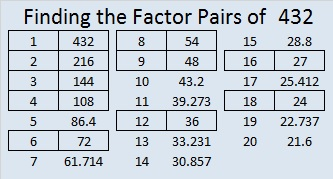# A Forest of 240 Factor Trees

### Factor Trees for 240:

Because 240 has so many factors, it is possible to make MANY different factor trees that create a forest of 240 factor trees. This post only contains eleven of those many possibilities. The two trees below demonstrate different permutations that can be made from the same basic tree. The mirror images of both, as well as mirror images of parts of either tree, would be other permutations.A good way to make a factor tree for a composite number is to begin with one of its factor pairs and then make factor trees for the composite numbers in that factor pair.

This forest of 240 factor trees is dedicated to Joseph Nebus. Read the comments to his post, You might also like, because, I don’t know why, to discover why I was inspired to create images of parts of this forest.

### Factors of 240:

• 240 is a composite number.
• Prime factorization: 240 = 2 x 2 x 2 x 2 x 3 x 5, which can be written 2⁴ x 3 x 5
• The exponents in the prime factorization are 4, 1 and 1. Adding one to each and multiplying we get (4 + 1)(1 + 1)(1 + 1) = 5 x 2 x 2 = 20. Therefore 240 has 20 factors.
• Factors of 240: 1, 2, 3, 4, 5, 6, 8, 10, 12, 15, 16, 20, 24, 30, 40, 48, 60, 80, 120, 240
• Factor pairs: 240 = 1 x 240, 2 x 120, 3 x 80, 4 x 60, 5 x 48, 6 x 40, 8 x 30, 10 x 24, 12 x 20, or 15 x 16
• Taking the factor pair with the largest square number factor, we get √240 = (√16)(√15) = 4√15 ≈ 15.492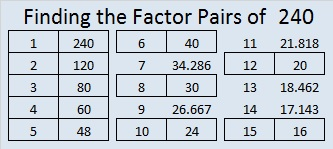### Sum-Difference Puzzle:

60 has six factor pairs. One of those factor pairs adds up to 17, and a different one subtracts to 17. Can you find those factor pairs to solve the first puzzle below?

240 has ten factor pairs. One of them adds up to 34, and another one subtracts to 34. If you can identify those factor pairs, then you can solve the second puzzle.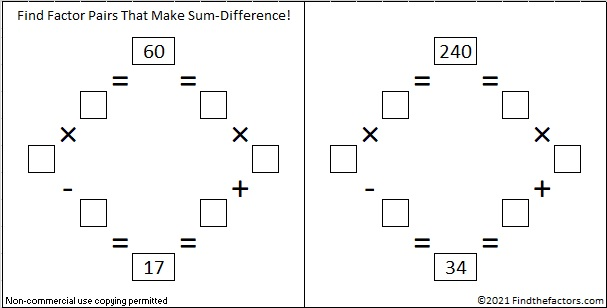The second puzzle is really just the first puzzle in disguise. Why would I say that?

### Another Fact about the Number 240:

2 + 4 + 6 + 8 + 10 + 12 + 14 + 16 + 18 + 20 + 22 + 24 + 26 + 28 + 30 = 240; that’s the sum of the first 15 even numbers.